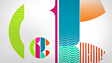An explanation of how to read numbers on a number line and how to speed up the process to be most efficient, demonstrated by the animated characters Star, Sky and Hutch. The first number line is read to find tens. Next, 58 is found on a number line using first the tens and then the units to find the number. A group of children form a human number line and expand the range of strategies which can be used for reading numbers on a number line. To find 78 it is faster to find 80 and then count back 2. Similarly, 63 is nearer to 60 than 70, so it is faster to find 60 in the tens first and then count on the units along the number line. Numbers ending in 5 are always halfway between the 10s. To find numbers in the hundreds, you can use exactly the same method as for smaller numbers.This clip is from:
Starship, Place Value
First broadcast:
10 January 2008

Can be used in a lesson on using number lines. The clip can be played to help pupils create their own number line (lower ability pupils could be given one already drawn and just have to fill in some blanks). Pupils can then be given random numbers and asked to quickly find and point it out on their number line. This can then be used to help pupils count in tens and then units as a process of chunking and partitioning, either in the same or in future lessons.